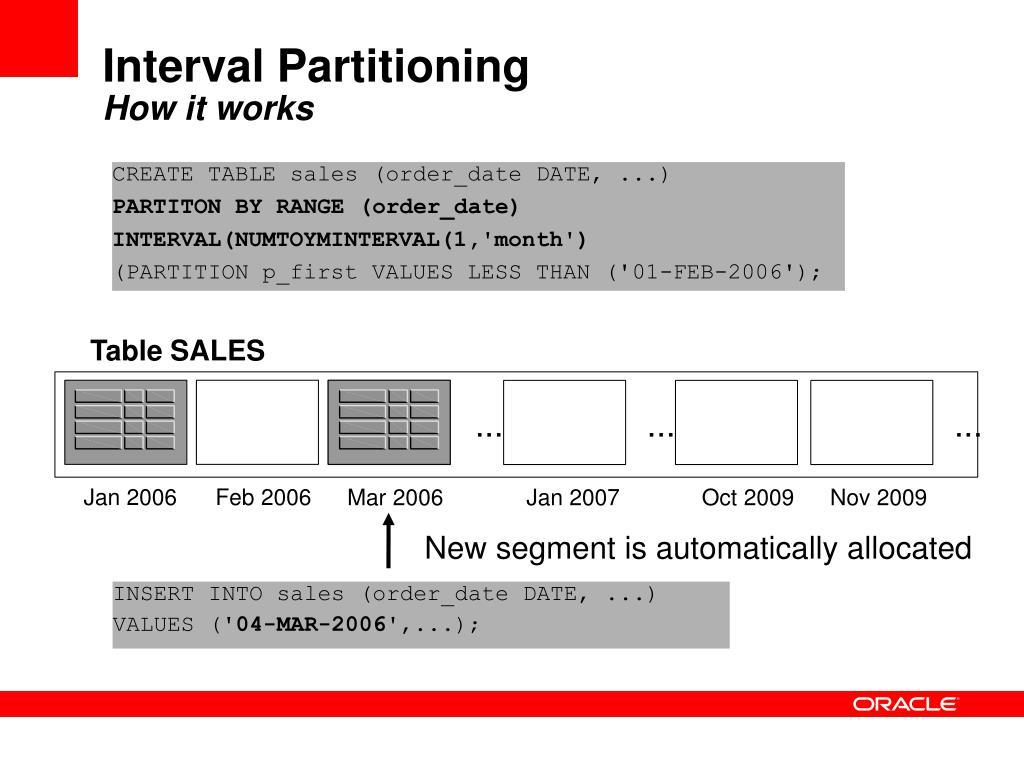# How to Change Existing Range Partitioned Table to Interval Partitioned Table and Interval Partitioned to Range Partitioned Table

Hi,

I will explain How to Change Existing Range Partitioned Table to Interval Partitioned Table and Interval Partitioned to Range Partitioned Table in this post.If you don’t know what is the Partitioning and What is the Range and Interval partitioning, read the following article.

Partitioning Types ( Range , List, Hash, Interval .. ) in Oracle Database

Interval Partitioning: This partitioning method is a new method used with Range partitioning that released in Oracle 11g. In the range partitioning method, new data was insert to the appropriate partition, but it gave the ORA-14400 error if it could not find the proper partition. This problem is solved automatically with Interval Partititoning. Interval Partitioning and Range Partitioning are used as follows.

If you are getting the following error frequently, then use the Interval partitioning.

`ORA-14400: inserted partition key does not map to any partition`

Interval partitioning table can be created like following. You can modify tablespace name and partitions name according to your need.

```SQL> Create table billing(customer_id number,
name varchar(50),
lastname varchar2(50),
bill_date date
)
PARTITION BY RANGE(bill_date)
INTERVAL(NUMTOYMINTERVAL(1,'YEAR'))
STORE IN (BILLING_TBS)
(
PARTITION Billing201901 VALUES LESS THAN(TO_DATE('01/01/2019','MM/DD/YYYY'))
);

```

Interval partitioning key column must be a DATE or NUMBER and this partitioning type cannot be used for index organized tables.

You cannot create domain indexes on Interval partitioning.

You cannot use the Interval partitioning for sub-partition because it is not supported.

Range partitioned table can be converted to Interval partitioning by using the following command.

`ALTER TABLE TABLE_NAME SET INTERVAL (interval value);`

NUMTOYMINTERVAL function converts number n to an INTERVAL YEAR TO MONTH and one of the following string values can be used;

‘YEAR’

‘MONTH’

For example;

If you want to convert Range partitioned table to Yearly Interval partitioned table, then use the following command.

`ALTER TABLE TABLE_YEARLY_PARTITIONED SET INTERVAL(NUMTOYMINTERVAL(1,'YEAR'));`

If you want to convert Range partitioned table to Monthly Interval partitioned table, then use the following command.

`ALTER TABLE TABLE_MONTHLY_PARTITIONED SET INTERVAL(NUMTOYMINTERVAL(1,'MONTH'));`

The NUMTODSINTERVAL function converts a number to an INTERVAL DAY TO SECOND literal.

For example:

```NUMTODSINTERVAL(180, 'DAY')
Result: '+000000180'

NUMTODSINTERVAL(1800, 'HOUR')
Result: '+000000075'

NUMTODSINTERVAL(18000, 'MINUTE')
Result: '+000000012'

NUMTODSINTERVAL(180000, 'SECOND')
Result: '+000000002'```

If you want to convert Range partitioned table to Daily Interval partitioned table, then use the following command.

`ALTER TABLE TABLE_DAILY_PARTITIONED SET INTERVAL NUMTODSINTERVAL(1,'day'));`

If you want to convert existing Interval partitioning to Range partitioning, you can use the following command.

`ALTER TABLE TABLE_NAME SET INTERVAL ();`

You can read the following post to learn how to list and analyze partitions of table.

How to List and Analyze Partitions of Table Order by High Value Column in Oracle Database

### Do you want to learn Oracle Database for Beginners, then read the following articles.

https://ittutorial.org/oracle-database-19c-tutorials-for-beginners/